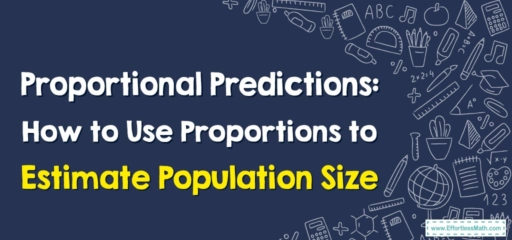# Proportional Predictions: How to Use Proportions to Estimate Population Size

Hello, math enthusiasts!

Today, we'll be talking about an incredibly practical use of math - using proportions to estimate population sizes. This technique is widely used in fields such as ecology, epidemiology, and market research, but it's also something you can apply in your daily life.## 1. Proportions: A Quick Refresher

A proportion is a mathematical statement showing that two ratios are equal. For instance, if there are $$5$$ apples and $$15$$ oranges, the ratio of apples to oranges is $$5:15$$, which simplifies to $$1:3$$. If you were to say, “For every apple, there are $$3$$ oranges,” you’d be expressing a proportion.

## 2. Using Proportions to Estimate Population Sizes

So, how can we use proportions to estimate population sizes? Suppose you want to estimate the number of fish in a lake. It’s not practical (or particularly nice for the fish) to try and count each one. Instead, you could catch a small sample of fish, mark them in a harmless way, and release them back into the lake. After giving them time to mix back into the population, you’d catch another sample. The proportion of marked fish in the second sample can give you a good estimate of the total fish population.

## Step-By-Step Guide to Using Proportions to Estimate Population Size

Here’s how you might use proportions to estimate population size, step by step:

### Step 1: Take a Sample and Mark

Catch a small sample from the population and mark or tag them. For instance, let’s say we catch and mark 50 fish from the lake.

### Step 2: Release and Resample

Release the marked sample back into the population. Wait for a period to let them mix back in. Then, take a new sample from the population. Let’s say we catch $$80$$ fish on our second go.

### Step 3: Count the Marked Sample in the Second Sample

Count the number of marked fish in your second sample. Suppose $$20$$ of our $$80$$ fish have marks.

### Step 4: Set Up a Proportion

Now you can set up a proportion. The ratio of marked fish to total fish in the second sample should be approximately equal to the ratio of marked fish to total fish in the entire population. This gives us:

$$\frac{20 \ (marked fish in second sample)}{80 \ (total fish in second sample)}$$ $$=$$ $$\frac{50 \ (marked fish in total)}{x \ (total fish in the lake)}$$

### Step 5: Solve for the Unknown

Solving this equation for $$x$$ gives us an estimate of the total fish population in the lake. Cross multiplying gives us $$20\times x = 4000$$, so $$x = \frac{4000}{20} = 200$$ fish in the lake.

And voila! We’ve used proportions to estimate the total population size.

Remember, this is an estimation method. The actual population size could be somewhat more or less than our estimate, especially with smaller or less well-mixed samples. However, this method still provides a valuable tool for population estimation in a wide range of fields.

So, keep honing your math skills, and who knows? You might be the next to make a significant statistical discovery in the real world!

This blog post introduces the concept of using proportions to estimate population sizes, providing a straightforward explanation along with a step-by-step guide to understanding and applying the concept.

### What people say about "Proportional Predictions: How to Use Proportions to Estimate Population Size - Effortless Math: We Help Students Learn to LOVE Mathematics"?

No one replied yet.

X
30% OFF

Limited time only!

Save Over 30%

SAVE $5 It was$16.99 now it is \$11.99